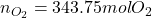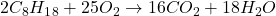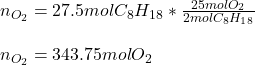## 1. The octane present in gasoline burns according to the following equation: 2 C8H18 + 25 O2 → 16 CO2 + 18 H2O

Question

1. The octane present in gasoline burns according to the following equation:

2 C8H18 + 25 O2 → 16 CO2 + 18 H2O

(a) How many moles of O2 are needed to react fully with 27.5 moles of octane?

in progress 0
5 months 2021-09-01T03:49:46+00:00 1 Answers 0 views 0Explanation:

Hello there!

In this case, according to the combustion of octane:We can see there is a 2:25 mole ratio of octane to oxygen; therefore, we can calculate the moles of oxygen via the following stoichiometric factor:Best regards!